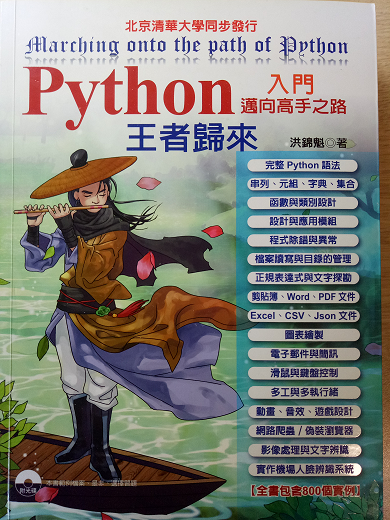4

pyautogui並非內建模組，

# 注意事項

``````pyautogui.PAUSE = 3
``````

# 一、滑鼠篇

## 取得滑鼠座標

``````import pyautogui
import time
width, height = pyautogui.size() # 取得螢幕寬度、高度
print(width, height)

#每隔一段時間取得滑鼠座標
for i in range(20):
time.sleep(1) # 暫停一秒
xloc, yloc = pyautogui.position() # 獲得滑鼠游標
print(xloc,yloc, end='\r', flush= True)
``````

## 移動滑鼠

1. 絕對位置
``````"""

"""

import pyautogui

x,y = 300,300
for i in range(3):
pyautogui.moveTo(x,y,duration=0.5)
pyautogui.moveTo(x+1200,y,duration=0.5)
pyautogui.moveTo(x+1200,y+400,duration=0.5)
pyautogui.moveTo(x,y+400,duration=0.5)
``````
1. 相對位置
``````"""

"""

import pyautogui

for i in range(3):
pyautogui.moveRel(300,0,duration=0.5)
pyautogui.moveRel(0,300,duration=0.5)
pyautogui.moveRel(-300,0,duration=0.5)
pyautogui.moveRel(0,-300,duration=0.5)
``````

## 按下滑鼠

`click()`可以讓滑鼠按下再放開，

``````import pyautogui
pyautogui.click(300, 400, button='right', duration=1)
``````

`click()`可以讓滑鼠按下再放開的動作，

# 二、鍵盤篇

``````import pyautogui
import time

print("請在5秒內開啟記事本並設為焦點視窗")
time.sleep(5) #程式暫停五秒，讓你有時間打開記事本
pyautogui.typewrite(['H','e','l','l','o'],0.1) #每隔0.1秒輸出一個字
``````

# 參考資料

1. (書本) Python入門邁向高手之路王者歸來/ 出版社：深石/ 作者： 洪錦魁